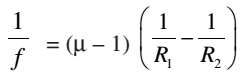# Lens Maker’s Formula and Magnification

The focal length of a lens depends on the radii of curvature of spherical surfaces. Focal length of a lens of larger radii of curvature will be more.

Focal length of a lens is smaller if the refractive index of its material is high.

Lens Maker’s Formula### Magnification

The magnification of a lens is defined as the ratio of the height of the image formed by the lens to the height of the object and is denoted by m.

m = I/O = v/u